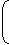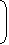# Aptitude - Surds and Indices

### Exercise :: Surds and Indices - General Questions

11.

 (243)n/5 x 32n + 1 = ? 9n x 3n - 1

 A. 1 B. 2 C. 9 D. 3n

Explanation:

Given Expression
 = (243)(n/5) x 32n + 1 9n x 3n - 1
 = (35)(n/5) x 32n + 1 (32)n x 3n - 1
 = (35 x (n/5) x 32n + 1) (32n x 3n - 1)
 = 3n x 32n + 1 32n x 3n - 1
 = 3(n + 2n + 1) 3(2n + n - 1)
 = 33n + 1 33n - 1
 = 3(3n + 1 - 3n + 1)   = 32   = 9.

12.

 1 + 1 = ? 1 + a(n - m) 1 + a(m - n)

A. 0
B.
 1 2
C. 1
D. am + n

Explanation:

1 + 1 =
1  +  11 + anam1 + aman
1 + a(n - m) 1 + a(m - n)

 = am + an (am + an) (am + an)

 = (am + an) (am + an)

= 1.

13.

If m and n are whole numbers such that mn = 121, the value of (m - 1)n + 1 is:

 A. 1 B. 10 C. 121 D. 1000

Explanation:

We know that 112 = 121.

Putting m = 11 and n = 2, we get:

(m - 1)n + 1 = (11 - 1)(2 + 1) = 103 = 1000.

14.xb(b + c - a) .xc(c + a - b) .xa(a + b - c) = ? xc xa xb

 A. xabc B. 1 C. xab + bc + ca D. xa + b + c

Explanation:

Given Exp.
 = x(b - c)(b + c - a) . x(c - a)(c + a - b) . x(a - b)(a + b - c)
 = x(b - c)(b + c) - a(b - c)  .  x(c - a)(c + a) - b(c - a)   .  x(a - b)(a + b) - c(a - b)
 = x(b2 - c2 + c2 - a2 + a2 - b2)  .   x-a(b - c) - b(c - a) - c(a - b)
 = (x0 x x0)
 = (1 x 1) = 1.

15.

 If x = 3 + 22, then the value ofx - 1is: x

 A. 1 B. 2 C. 22 D. 33

Explanation:x - 12 = x + 1 - 2 x x

 = (3 + 22) + 1 - 2 (3 + 22)

 = (3 + 22) + 1 x (3 - 22) - 2 (3 + 22) (3 - 22)

= (3 + 22) + (3 - 22) - 2

= 4.x - 1= 2. x